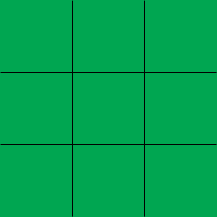SEARCH HOMEMath Central Quandaries & QueriesQuestion from Victor: 6200 sq. ft.into yardsHi Victor,

Do you mens square feet into square yards? if so then there are 3 feet on a yard so a square that measures 1 yard on each side can be covered with 9 squares that measure 1 foot on each side.Thus 1 square yard = 9 square feet and hence 6200 square feet = 6200/9 = 688.89 square yards.

PennyMath Central is supported by the University of Regina and The Pacific Institute for the Mathematical Sciences.